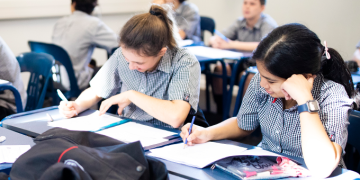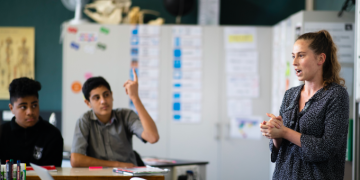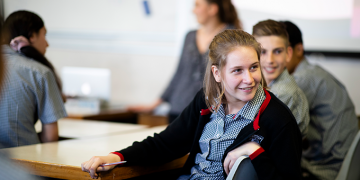##### Resources

Explore our wide range of resources to build financial capability knowledge and skills.##### Teacher hub

Customise your financial capability learning programme here.##### Student hub

Start your journey to become financially capable here.Sorted in Schools is a free financial capability programme fully aligned to the National Curriculum.

# Maths resources

###### This resource encourages students to apply their maths skills and knowledge within the context of financial sustainability. Students explore investments/whakangao, Insurance/inihua, KiwiSaver and retirement/whakatā.

Exploring financial sustainability through maths and statistics is an integrated, cross-curricular resource and can be used in multiple ways. Related resources are available for health and social sciences.

There are a range of resources to choose from, so schools and teachers can design programmes that allow:

• Students to work at their own pace using a student planner
• Teachers to design a programme that suits department, faculty or whole-school planning over a few weeks or a term. This will vary from school to school.

### Mathematics and statistics achievement objectives

In a range of meaningful contexts, students will be engaged in thinking mathematically and statistically. They will solve problems and model situations that require them to:

##### Number and Algebra, Level Four
• Understand addition and subtraction of fractions, decimals and integers
• Find fractions, decimals and percentages of amounts expressed as whole numbers, simple fractions and decimals
• Apply simple linear proportions, including ordering fractions
• Know the equivalent decimal and percentage forms for everyday fractions
• Know the relative size and place-value structure of positive and negative integers, and decimals to three places
• Form and solve simple linear equations
##### Number and Algebra, Level Five
• Reason with linear proportions
• Understand operations on fractions, decimals, percentages and integers
• Use rates and ratios
• Know commonly used fraction, decimal and percentage conversions
• Know and apply standard form, significant figures, rounding and decimal-place value
• Form and solve linear and simple quadratic equations
##### Geometry and Measurement, Level Four
• Convert between metric units, using whole numbers and commonly used decimals
• Use side or edge lengths to find the perimeters and areas of rectangles, parallelograms and triangles, and the volumes of cuboids.
##### Geometry and Measurement, Level Five
• Select and use appropriate metric units for length, area, volume and capacity, weight (mass), temperature, angle and time, with the awareness that measurements are approximate
• Convert between metric units using decimals
• Deduce and use formulae to find the perimeters and areas of polygons and the volumes of prisms
• Find the perimeters and areas of circles and composite shapes and the volumes of prisms, including cylinders